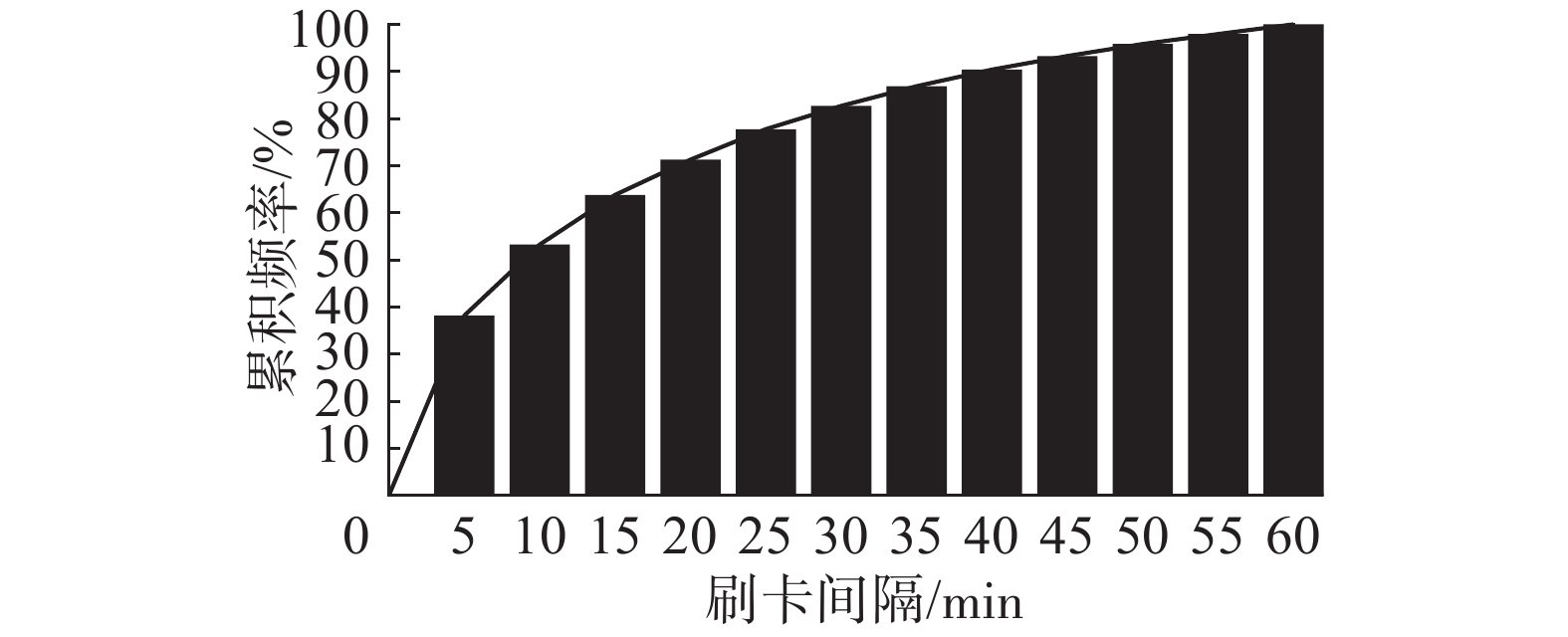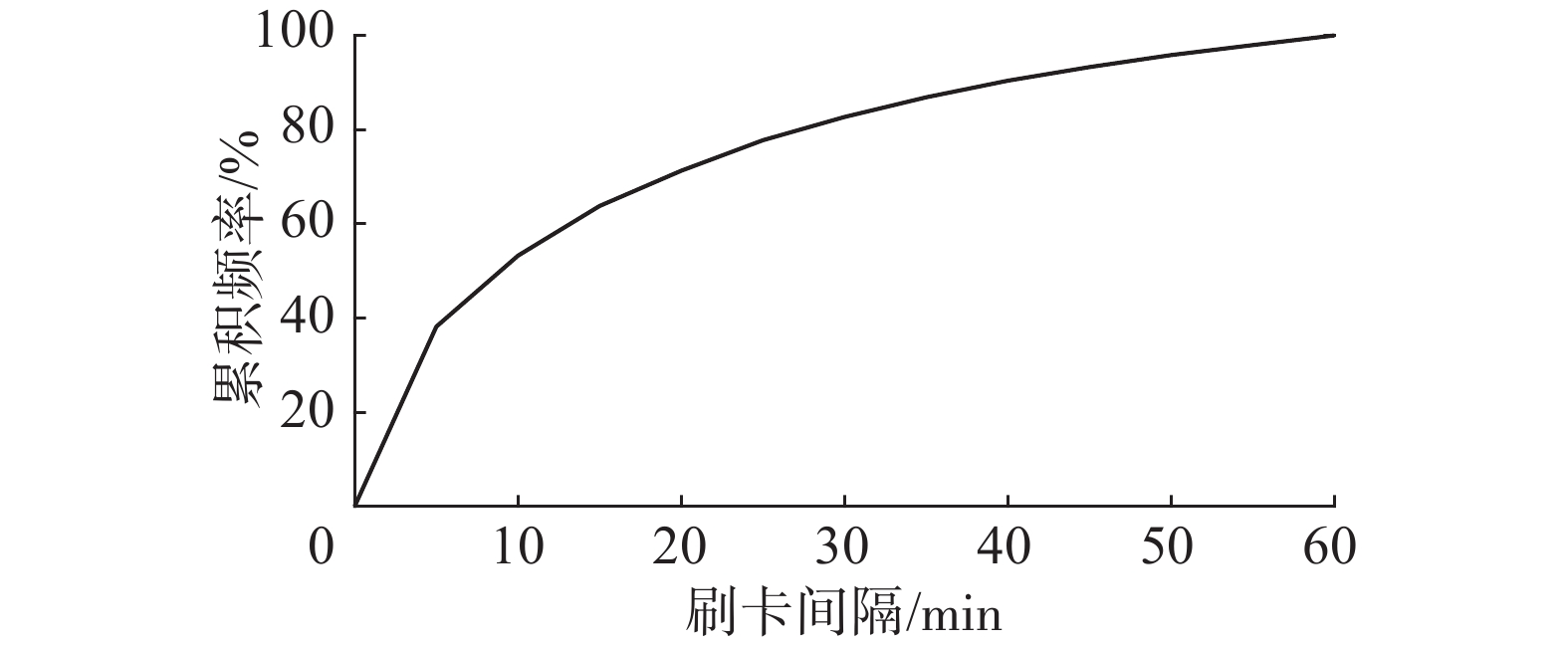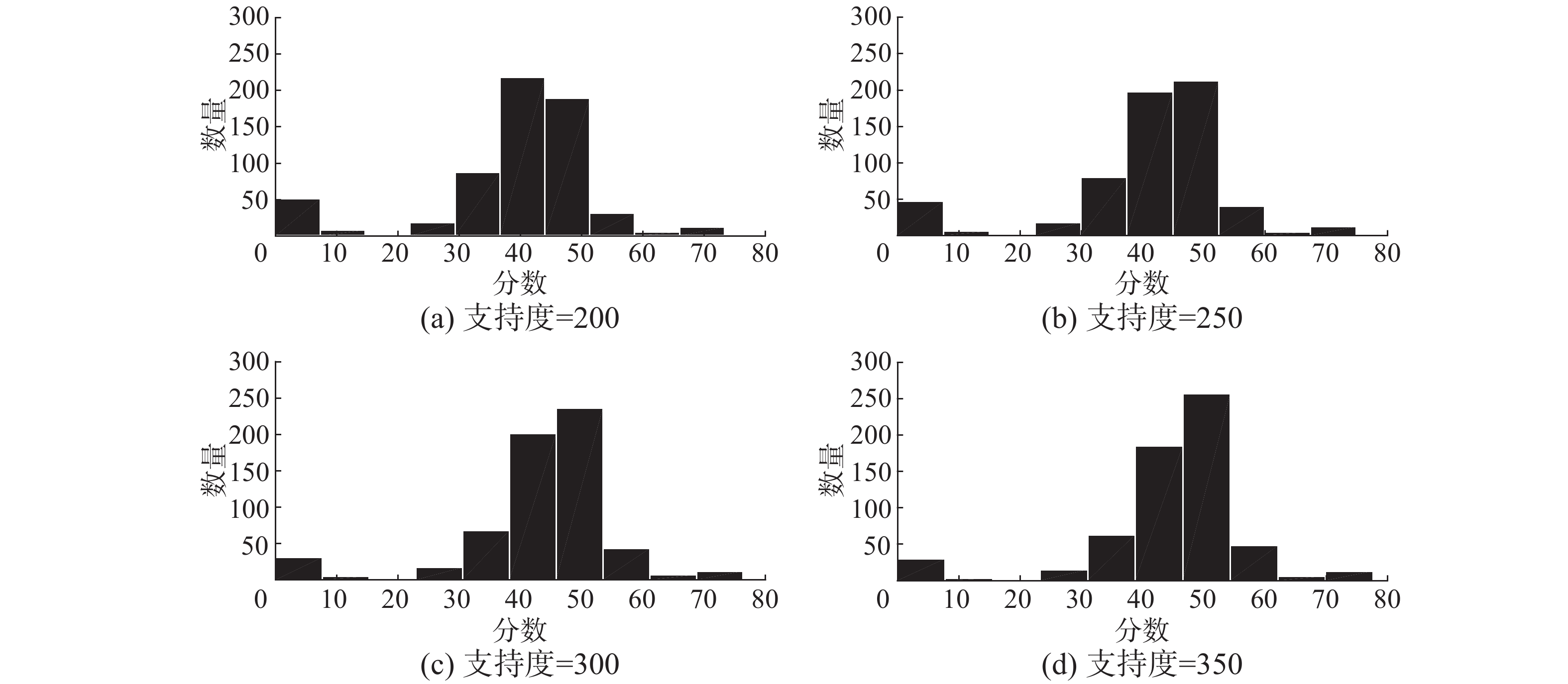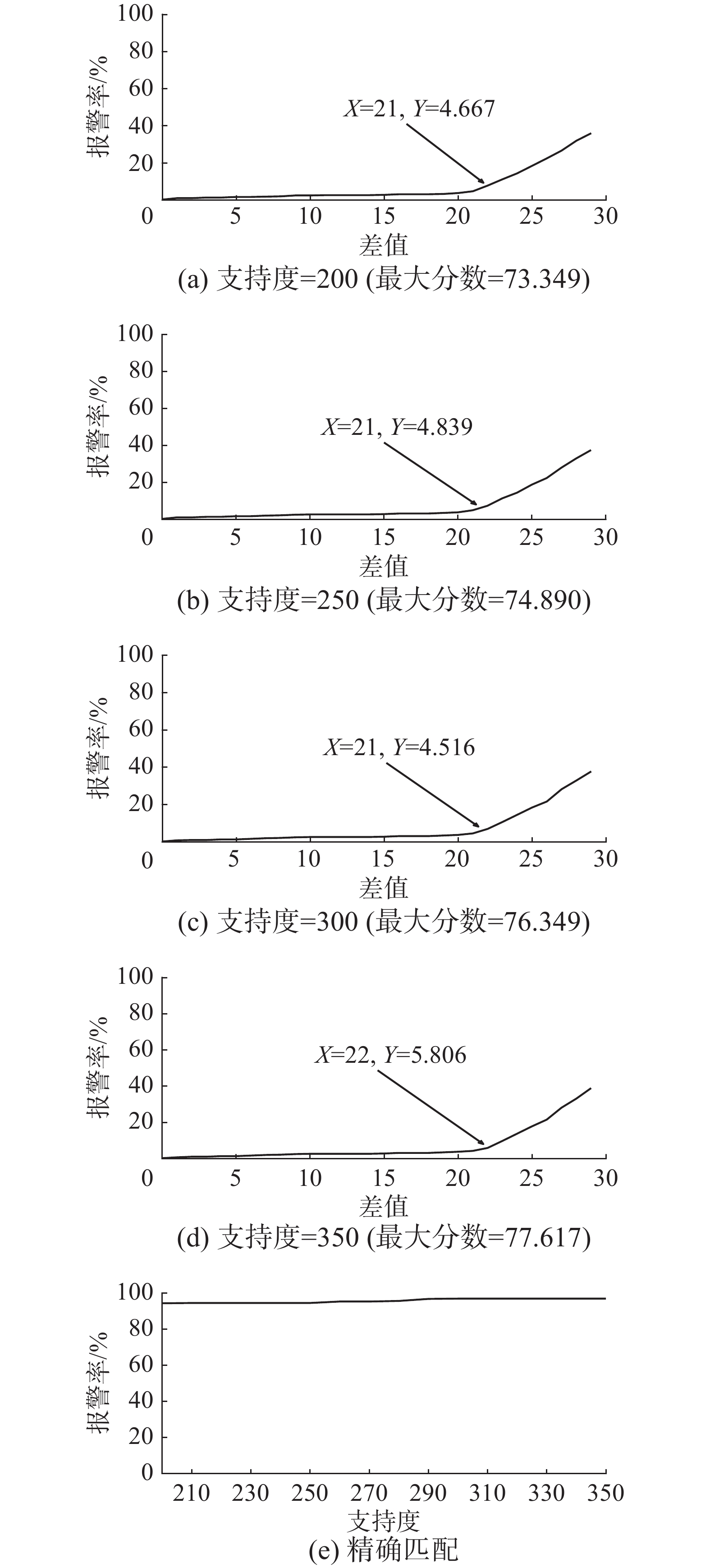﻿ 基于门禁日志挖掘的内部威胁异常行为分析
«上一篇文章快速检索 高级检索

 智能系统学报2017, Vol. 12Issue (6): 781-789  DOI: 10.11992/tis.2017060410

### 引用本文WANG Peichao, ZHOU Yun, ZHU Cheng, et al. Analysis on abnormal behavior of insider threats based on accesslog mining[J]. CAAI Transactions on Intelligent Systems, 2017, 12(6): 781-789. DOI: 10.11992/tis.201706041.### 文章历史

Analysis on abnormal behavior of insider threats based on accesslog mining
WANG Peichao, ZHOU Yun, ZHU Cheng, HUANG Jincai, ZHANG Weiming
Key Laboratory of Information System Engineering, National University of Defense Technology, Changsha 410072, China
Abstract: Using an access control system is an important method of guarding key places, and it can effectively prohibit the entry of unauthorized users. However, many recent cases indicate that threats to key places mostly come from insiders. To address this challenge, this paper proposes a method for analyzing the abnormal behavior of insider threats based on accesslog data mining. First, the PrefixSpan algorithm is used to extract normal behavior sequences; then, the anomaly scores of the access sequences are calculated. Finally, the abnormal sequences are identified according to a threshold determined by decision makers. Experiments on real access data show that this method can decrease high false alarm rates caused by an exact match when there is limited data and can also effectively reveal abnormal behavior by insiders. Therefore, this method provides a new approach for enhancing the protection of key places.
Key words: access control system    accesslog mining    insider threat detection    analysis on abnormal behavior

1 相关研究

2 问题描述 2.1 路径序列数据

2.2 正常路径序列数据

2.3 序列异常度分数

3 异常序列挖掘

3.1 序列差异分数 3.1.1 正常行为序列库建立

3.1.2 相对编辑距离计算

 ${R_{\rm ED}}\left( {q_i^t,q_j^n} \right) = \frac{{{\rm{ED}}\left( {q_i^t,q_j^n} \right)}}{{{\rm Max}\left( {\left| {q_i^t\left| , \right|q_j^n} \right|} \right)}}$ (1)

3.1.3 相对支持度计算

 ${R_{\rm sup}}\left( {q_j^n} \right) = \frac{{\left. {{\rm log}({C_{\rm sup}}\left( {q_j^n} \right)} \right)}}{{\left. {\log ({\rm Max}{_{\rm sup}}} \right)}}$ (2)

3.1.4 序列差异分数计算

1）当相对编辑距离计算结果中存在0时，意味着当前行为序列与正常行为序列库中的序列存在完全一致的情况，因此此时序列差异分数为0；

2）当相对编辑距离计算结果中不存在0时，意味着当前行为序列与正常行为序列库中的序列不存在完全一致的情况，这时考虑当前行为序列与正常行为序列库中所有序列的整体差别，对计算出的多个得分求平均值，从而得到该行为序列偏离正常行为序列的总体程度：

 {\rm score_1}\left( {q_i^t,{q^n}} \right) = \left\{ {\begin{aligned}& 0, {\exists {R_{\rm ED}}\left( {q_i^t,q_j^n} \right) = 0}\\& {{\rm mean}(\mathop \sum \limits_{j = 1}^{{M_{{\rm{min}}\_{\rm sup}}}} 100 {R_{\rm sup}}\left( {q_j^n} \right) {R_{\rm ED}}\left( {q_i^t,q_j^n} \right))}, {\forall {R_{\rm ED}}\left( {q_i^t,q_j^n} \right) \ne 0}\end{aligned}} \right. (3)

3.2 时间异常分数 3.2.1 时间规则

1）异常时间段进入：用户在非正常时间段进入某地。

2）刷卡间隔过短：两次刷卡时间间隔过于短暂，异于平常。

3.2.2 时间分数计算

 \begin{aligned} {\rm score_2}\left( {q_i^t} \right) = \qquad \qquad \qquad \qquad \quad \\ 100 \times \left( {\displaystyle\frac{{\mathop \sum \limits_{k = 1}^{{N_i} - 1} \left( {1 - f\left( {\Delta {t_k}} \right)} \right)}}{{{N_i} - 1}} + \displaystyle\frac{{\left| {{\rm Min}\left( {0,{t_i} - {\rm threshold}} \right)} \right|}}{{\left| {\rm threshold} \right|}}} \right) \times \displaystyle\frac{1}{2}\end{aligned} (4)

3.3 序列异常度分数 3.3.1 序列异常度分数计算

 ${\rm score}\left( {q_i^t} \right) = \alpha \cdot {\rm score_1}\left( {q_i^t,{q^n}} \right) + \left( {1 - \alpha } \right) \cdot {\rm score_2}\left( {q_i^t} \right)$ (5)

3.3.2 异常路径行为发现

3.3.3 序列异常度分数计算示例表 1 测试用正常行为序列库 Tab.1 Testing normal sequences library

 ${\rm score}{_{1a}} = \frac{{\rm log\left( {15} \right)}}{{\rm log\left( {19} \right)}} \times \frac{2}{5} \times 100 = 36.79$
 ${\rm score}{_{1b}} = \frac{{\rm log\left( 3 \right)}}{{\rm log\left( {19} \right)}} \times \frac{2}{4} \times 100 = 18.66$
 ${\rm score}{_{1c}} = \frac{{\rm log\left( {19} \right)}}{{\rm log\left( {19} \right)}} \times \frac{2}{3} \times 100 = 66.67$
 ${\rm score}{_1} = \frac{{\left( {36.79 + 18.66 + 66.67} \right)}}{3} = 40.71$

 ${\rm scoer_2} \!=\! 100 \times \left[ {\frac{{\left( {1 \!-\! f\left( {32.1} \right)} \right) \!+\! \left( {1 \!-\! f\left( {4.1} \right)} \right)}}{2} \!+\! 0} \right] \! \times \! \frac{1}{2} \!=\! 18.33$

 ${\rm score} = 40.71 \times 0.5 + 18.33 \times 0.5 = 29.52$

4 实验结果及分析

4.1 数据预处理表 2 门禁数据属性说明 Tab.2 Instruction on attributes of access control system

4.2 正常路径行为模型建立表 3 正常路径序列模式集 Tab.3 Normal set of road sequences图 1 刷卡间隔与累积频率曲线 Fig.1 Curve of swiping card interval and cumulative frequency

 $f\left( {\Delta t} \right) = 0.172 \; 6 \times {\left( {2.11 \times \Delta t} \right)^{0.370 \; 8}}$

R2为0.983，证明该函数对数据有较好的拟合效果。图 2 刷卡间隔与累积频率拟合曲线 Fig.2 Fitted curve of swiping card interval and cumulative frequency
4.3 异常行为发现及对比图 3 序列异常度分数分布 Fig.3 Distribution of score of sequence’s abnormal degree图 4 部门A报警率曲线 Fig.4 Department A’s curve of alarm rate

p0p32p30p48p32〉：当天的刷卡记录中只有从办公室之间的刷卡记录，没有出现正门的刷卡记录。

p32p32p4，…，p48p32p32〉：平日刷卡记录极少的p32在当天出现了大量的刷卡记录。

5 结束语

  杨荣秀. 基于指纹识别技术的智能小区门禁系统的设计[J]. 科技与企业, 2016(5): 88-90. YANG Xiurong. Design of intelligent community access control system based on fingerprint identification technique[J]. Technology and enterprise, 2016(5): 88-90. (0)  李海青, 孙哲南, 谭铁牛, 等. 虹膜识别技术进展与发展趋势[J]. 信息安全研究, 2016, 2(1): 40-43. LI Haiqing, SUN Zhenan, TAN Tieniu, et al. Progress and trends in iris recognition[J]. Journal of information security research, 2016, 2(1): 40-43. (0)  FERRAIOLO D F, KUHN R. Role based access control[C]//Proceedings of the 15th NIST-NCSC National Computer Security Conference. Baltimore, Maryland, 1992: 554-563. (0)  MATT B, SOPHIE E, SEAN P, et al. We have met the enemy and he is us[C]//New Security Paradigms Workshop. Lake Tahoe, USA, 2008: 1-11. (0)  JIAN Pei, HAN Jiawei, BEHZAD M, et al. PrefixSpan: mining sequential patterns efficiently by prefix-projected pattern growth[C]//20th International Council for Open and Distance Education World Conference on Open Learning and Distance Education. Heidelberg, Germany, 2001: 215-224. (0)  ANTONIO L, SIMON F, ZHUNAG Yan. A logical model for detecting irregular actions in physical access[C]// IEEE conference on database and expert systems applications. [S.l.], 2007: 560-564. (0)  DAVIS M, LIU W, MILLER P, et al. Detecting anomalise in graphs with numeric labels[C]//ACM Conference on Information and Knowledge Management. Glasgow, United Kingdom, 2011: 1197-1202. (0)  GOKHAN K, DUC L, TING X, et al. Ettu: analyzing query intents in corporate databases[C]//Proceedings of the 25th International Conference Companion on World Wide Web. Montreal, Canada, 2016: 463-466. (0)  TABISH R, IOANNIS A, JASON R. A new take on detecting insider threats: exploring the use of hidden markov models[C]//Proceedings of the 22nd International Conference on Intelligent User Interfaces Companion. Limassol, Cyprus, 2016: 47-56. (0)  TED E S, DAVID A B, THOMAS G D, et al. Detecting insider threats in a real corporate database of computer usage activity[C]//Proceedings of the 19th ACM SIGKDD International Conference on Knowledge Discovery and Data Mining. Chicago, USA, 2013: 1393-1401. (0)  王怀宝, 郭江利. 基于跟踪轨迹的徘徊行为分析[J]. 计算机与数字工程, 2016, 44(5): 843-846. WANG Huaibao, GUO Jiangli. Wandering behavior analysis based on trajectory[J]. Computer and digital engineering, 2016, 44(5): 843-846. (0)  邹一波, 陈一民. 基于运动标签的异常行为检测算法[J]. 计算机应用与软件, 2015, 5: 238-240, 266. ZOU Yibo, CHEN Yimin. Anomalous behaviors detection algorithm based on motion label[J]. Computer applications and software, 2015, 5: 238-240, 266. (0)  HAN Jiawei, MICHELINE K, PEI Jian. Data mining concepts and techniques[M]. 3版. 北京: 机械工业出版社: 2016: 355-356. (0)  BOSTJAN K, ERIK D, TEA T, et al. A probabilistic risk analysis for multimodal entry control[J]. Expert systems with applications, 2011, 38(6): 6696-6704. (0)  MICHAEL D, WEIRU L, PAUL M. Detecting anomalies in graphs with numeric labels[J]. ACM conference on information and knowledge management, 2011(10): 1197-1202. (0)  胡向东, 韩恺敏, 许宏如. 智能家居物联网的安全性设计与验证[J]. 重庆邮电大学学报:自然科学版, 2016, 26(2): 171-176. HU Xiangdong, Han Kaimin, XU Hongru. Design and implementation of security-focused intelligent household Internet of things[J]. Journal of Chongqing university of posts and telecommunications: natural science edition, 2016, 26(2): 171-176. (0)  胡向东, 唐飞. 智能家居门禁系统的安全控制方法[J]. 重庆邮电大学学报: 自然科学版, 2016, 28(6): 863-869. HU Xiangdong, TANG Fei. Secure control methods of the entrance guard system for smart home[J]. Journal of Chongqing university of posts and telecommunications: natural science edition, 2016, 28(6): 863-869. (0)  王菲. 数据挖掘在图书馆用户行为分析上的应用研究[D]. 上海: 上海交通大学, 2013: 26-49. WANG Fei. Data mining applied in the library user behavior analysis[D]. Shanghai: Shanghai Jiao Tong University, 2013: 26-49. (0)  郑伟平, 言专艺, 唐晓红. 电子门禁数据挖掘与应用方法[J]. 警察技术, 2015, 6: 47-50. ZHENG Weiping, YAN Zhuanyi, TANG Xiaohong. Access control data mining and application methods[J]. Police technology, 2015, 6: 47-50. (0)  史殿习, 李寒, 杨若松, 等. 用户日常频繁行为模式挖掘[J]. 国防科技大学学报, 2017, 39(1): 74-80. SHI Dianxi, LI Han, YANG Ruosong, et al. Mining user frequent behavior patterns in daily life[J]. Journal of national university of defense technology, 2017, 39(1): 74-80. (0)  顾兆军, 安一然, 刘飞. 基于航站楼门禁日志挖掘的物理入侵检测技术[J]. 计算机应用与软件, 2015, 32(11): 317-320, 324. GU Zhaojun, AN Yiran, LIU Fei. Physical intrusion detection technology based on terminal buildings access log mining[J]. Computer applications and software, 2015, 32(11): 317-320, 324. (0)  陈卓, 杨炳儒, 宋威, 等. 序列模式挖掘综述[J]. 计算机应用研究, 2008, 25(7): 1960-1964. CHEN Zhuo, YANG Bingru, SONG Wei, et al. Survey of sequential pattern mining[J]. Application research of computers, 2008, 25(7): 1960-1964. (0)  HAN Jiawei, PEI Jian, BEHZAD M, et al. FreeSpan: frequent pattern-projected sequential pattern mining[C]//Proceedings of the 6th ACM SIGKDD International Conference on Knowledge Discovery and Data Mining. New York, USA, 2000: 355-359. (0)